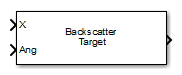•Libraries:
Phased Array System Toolbox / Environment and Target

## Description

The Backscatter Radar Target block models the monostatic radar reflections of nonpolarized electromagnetic signals from a target. Target model includes all four Swerling target fluctuation models and non-fluctuating model. You can model several targets simultaneously by specifying multiple radar cross-section (RCS) matrices.

## Ports

### Input

expand all

Narrowband nonpolarized signal, specified as an N-by-1 complex-valued vector or an N-by-M complex-valued matrix. The quantity N is the number of signal samples and M is the number of signals reflecting from the target. Each column corresponds to an independent signal incident at a different reflecting angle.

The size of the first dimension of the input matrix can vary to simulate a changing signal length. A size change can occur, for example, in the case of a pulse waveform with variable pulse repetition frequency.

Data Types: `double`

Incident signal direction, specified as a 2-by-1 real-valued column vector or a 2-by-M real-valued column matrix. M is the number of signals reflecting from the target. Each column of `Ang` specifies the incident direction of the corresponding signal in the form of an `[AzimuthAngle;ElevationAngle]` pair. Units are degrees. The number of columns in `Ang` must match the number of independent signals in `X`.

Example: `[30;45]`

Data Types: `double`

Switch to update RCS fluctuation model values, specified as `false` or `true`. When `Update` is `true`, the RCS value is updated. If `Update` is `false`, the RCS remains unchanged.

#### Dependencies

To enable this port, set the Fluctuation model drop-down menu to `Swerling1`, `Swerling2`, `Swerling3`, or `Swerling4`.

### Output

expand all

Narrowband nonpolarized signal, specified as an 1-by-M complex-valued vector or a N-by-M complex-valued matrix. Each column contains an independent signal reflected from the target.

The quantity N is the number of signal samples and M is the number of signals reflecting off the target. Each column corresponds to a different reflecting angle.

The output port contains signal samples arriving at the signal destination within the current input time frame. When the propagation time from source to destination exceeds the current time frame duration, the output does not contain all contributions from the input of the current time frame.

## Parameters

expand all

Specify the azimuth angles used to define the angular coordinates of the RCS pattern (m^2) parameter. Specify azimuth angles as a length P vector. Units are degrees. P must be greater than two. This parameter determines the incident azimuthal arrival angle of any element of the cross-section patterns.

Data Types: `double`

Specify the elevation angles used to define the angular coordinates of the RCS pattern (m^2) parameter. Specify elevation angles as a length Q vector. Units are degrees. Q must be greater than two. This parameter determines the incident elevation arrival angle of any element of the cross-section patterns.

Radar cross-section pattern, specified as a Q-by-P real-valued matrix or a Q-by-P-by-M real-valued array.

• Q is the length of the vector in the Elevation angles (deg) parameter.

• P is the length of the vector in the Azimuth angles (deg) parameter.

• M is the number of target patterns. The number of patterns corresponds to the number of signals passed into the input port `X`. You can, however, use a single pattern to model multiple signals reflecting from a single target.

You can, however, use a single pattern to model multiple signals reflecting from a single target. Pattern units are square-meters.

Pattern units are square-meters.

Specify the statistical model of the target as either `Nonfluctuating`, `Swerling1`, `Swerling2`, `Swerling3`, or `Swerling4`. When you set this parameter to a value other than `Nonfluctuating`, you then set radar cross-sections parameters using the `Update` input port.

Signal propagation speed, specified as a real-valued positive scalar. The default value of the speed of light is the value returned by `physconst('LightSpeed')`. Units are in meters per second.

Example: `3e8`

Data Types: `double`

Carrier frequency of the signal that reflects from the target, specified as a positive scalar. Units are in hertz.

Data Types: `double`

Block simulation, specified as `Interpreted Execution` or `Code Generation`. If you want your block to use the MATLAB® interpreter, choose `Interpreted Execution`. If you want your block to run as compiled code, choose ```Code Generation```. Compiled code requires time to compile but usually runs faster.

Interpreted execution is useful when you are developing and tuning a model. The block runs the underlying System object™ in MATLAB. You can change and execute your model quickly. When you are satisfied with your results, you can then run the block using ```Code Generation```. Long simulations run faster with generated code than in interpreted execution. You can run repeated executions without recompiling, but if you change any block parameters, then the block automatically recompiles before execution.

This table shows how the Simulate using parameter affects the overall simulation behavior.

When the Simulink® model is in `Accelerator` mode, the block mode specified using Simulate using overrides the simulation mode.

Acceleration Modes

 Block Simulation Simulation Behavior `Normal` `Accelerator` `Rapid Accelerator` `Interpreted Execution` The block executes using the MATLAB interpreter. The block executes using the MATLAB interpreter. Creates a standalone executable from the model. `Code Generation` The block is compiled. All blocks in the model are compiled.

 Block Parameter:`SimulateUsing` Type:enum Values:```Interpreted Execution```, `Code Generation` Default:```Interpreted Execution```